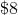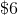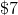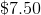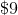# ISEE Lower Level Math : How to identify the parts of a list

## Example Questions

### Example Question #11 : How To Identify The Parts Of A List

What is the mean of the following set of numbers?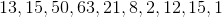Explanation:

The mean represents the average of a set of values. To find the mean, or average, first find the sum of the set of numbers.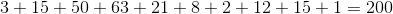Then, divide the sum by however many numbers are in the set. In this set there are ten numbers, so we will divide two hundred by ten.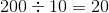Therefore, the mean is.

### Example Question #12 : How To Identify The Parts Of A List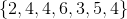What is the mode of this data set?Explanation:

The mode of a set is the number that occurs most often. In this set, it is 4.

### Example Question #13 : How To Identify The Parts Of A List

Which tool is best to measure the length of your bed?

Yardstick

Compass

Scale

Calculator

Ruler

Yardstick

Explanation:

Since the length of your bed is probably somewhere around 6 feet, the best tool to use is a yardstick. A ruler would require more measurements, and therefore, leave more room for error.

### Example Question #14 : How To Identify The Parts Of A List

Which answer choice descirbes the numbers in this set?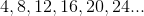Multiples of four

Odd numbers

Prime numbers

Factors of eight

None of these

Multiples of four

Explanation:

The set contains multiples of four.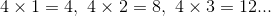Odd numbers are not divisible by two, and prime numbers have no factors other than the number itself and one. None of the numbers in this set are odd, nor are they prime. Four is a factor of eight, but none of the other numbers in the set are factors of eight.

### Example Question #15 : How To Identify The Parts Of A List

On a quiz of ten questions, the students in Mr. Becker's class earned the following scores: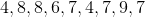What is the mode of their scores?Explanation:

The mode is the number that occurs most often in a set.The score of 7 occurs three times in this set, the most of any other score. Because it occurs most frequently, the mode is 7.

### Example Question #16 : How To Identify The Parts Of A List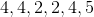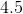Explanation:

The mode is the number that occurs most often in a set.The number that appears the most is 4, meaning that it must be the mode.

### Example Question #17 : How To Identify The Parts Of A List

The pet store is selling turtles that have the following lengths: 3 inches, 5 inches, 3 inches, 3 inches, 4 inches, 5 inches, 9 inches. What is the median of the turtle lengths?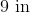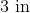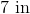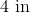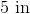Explanation:

When the lengths of the turtles are ordered from smallest to largest, the lengths are ordered as such:

3 inches, 3 inches, 3 inches, 4 inches, 5 inches, 5 inches, 9 inches

The median is the middle of the set. Given that there are 7 turtles, the 4th turtle would be in the middle. Since the 4th turtle is 4 inches long, that is the median.

### Example Question #18 : How To Identify The Parts Of A List

Lisa is an iceskater and was scored on a scale of 1 to 6 by a panel of judges. Her scores were: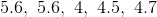What was the mode of her scores?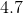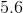Explanation:

The mode is the number that occurs most often in a set.Given that 5.6 was the score that was given more than any other (twice), it is the mode.

### Example Question #19 : How To Identify The Parts Of A List

Which of the following could be the value ofin the set?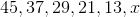Explanation:

Every subsequent number in this set is 8 less than the preceding number.

8 less than 13 is 5, which is therefore the correct answer.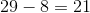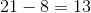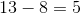### Example Question #20 : How To Identify The Parts Of A List

Lisa buys seven pairs of earrings. They cost the following amounts: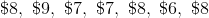What is the mode?